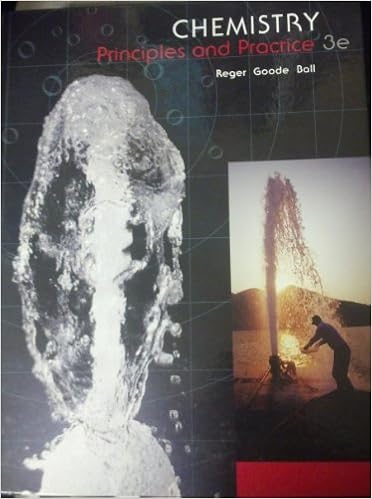# In these cases for example lih nah and cah 2 its

• Notes
• UltraLightningCobra8457
• 24

This preview shows page 14 - 17 out of 24 pages.

##### We have textbook solutions for you!
The document you are viewing contains questions related to this textbook.The document you are viewing contains questions related to this textbook.
Chapter 2 / Exercise 2.49
Chemistry: Principles and Practice
Reger ChemExpert Verified
In these cases (for example, LiH, NaH, and CaH 2 ), its oxidation number is -1. 5. Fluorine has an oxidation number of -1 in all its compounds. Other halogens (Cl, Br, and I) have negative oxidation numbers when they occur as halide ions in their compounds. When combined with oxygen-for example in oxoacids and oxoanions, they have positive oxidation numbers. 6. In a neutral molecule, the sum of the oxidation numbers of all the atoms must be zero. In a polyatomic ion, the sum of oxidation numbers of all the elements in the ion must be equal to the net charge of the ion. For example, in the ammonium ion, NH 4 + , the oxidation number of N is 3- and that of H is +1. Thus the sum of the oxidation numbers is -3 + 4(+1) = +1, which is equal to the net charge of the ion. Activity Series of Select Elements: (Least Active) Au, Hg, Ag, Cu, H 2 , Pb, Sn, Ni, Cd, Fe, Zn, Cr, Al, Mg, Na, Ca, K (Most Active) Diatomic Elements: H 2 , N 2 , F 2 , O 2 , I 2 , Cl 2 , Br 2 (Pneumonic for memorization: H ave N o F ear O f I ce C o l d B eve r ages)
##### We have textbook solutions for you!
The document you are viewing contains questions related to this textbook.The document you are viewing contains questions related to this textbook.
Chapter 2 / Exercise 2.49
Chemistry: Principles and Practice
Reger ChemExpert Verified
21 Redox Equation Balancing Ion-Electron Method In acid solution: 1. Write two half-reactions, one representing the oxidation, and the other the reduction, that occur in the reaction. It is not necessary to know which is which at this point. 2. In each half-reaction, balance all atoms except hydrogen and oxygen. 3. Balance oxygen atoms in each half-reaction by adding one H 2 O molecule for each oxygen atom needed. Never use O 2 , OH - , or any other form of oxygen. 4. Balance the hydrogen atoms by adding hydrogen ions, H + . Never use H 2 , OH - , or any other form of hydrogen. 5. Balance the charges by adding the proper number of electrons (e - ). If steps 1-4 have been done properly, electrons will be added to the left side of one half-reaction and to the right side of the other. 6. Multiply each half-reaction by the appropriate number so that the two half-reactions have the same number of electrons. Add the half-reactions, and cancel the electrons (they must cancel) . Also cancel all common ions and molecules. Simplify the coefficients of the equation if possible. If reaction takes place in basic solution: 7. In addition to steps 1-6, add one OH - ion for each H + ion to both sides of the equation in step 6. Combine the H + and the OH - ions on one side of the reaction into H 2 O molecules. Cancel H 2 O molecules that appear on both sides of the equation, and simplify if possible. If the redox reaction does not take place in acidic or basic solution, then you can usually solve the problem by inspection. Just make sure that both atoms and charges are balanced.
22 Base Triplets and Associated Amino Acids Second Base First Base U C A G Third Base U UUU Phe UCU Ser UAU Tyr UGU Cys U UUC UCC UAC UGC C UUA Leu UCA UAA Termination UGA Termination A UUG UCG UAG Termination UGG Trp G C CUU Leu CCU Pro CAU His CGU Arg U CUC CCC CAC CGC C CUA CCA CAA Gln CGA A CUG CCG CAG CGG G A AUU Ile ACU Thr AAU Asn AGU Ser U AUC ACC AAC AGC C AUA ACA AAA Lys AGA Arg A AUG Met ACG AAG AGG G G GUU Val GCU Ala GAU Asp GGU Gly U GUC GCC GAC GGC C GUA GCA GAA Glu GGA A GUG GCG GAG GGG G
•••